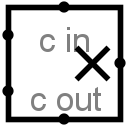#Multiplier

 Library: Arithmetic Introduced: 2.0 Beta 20 Appearance:## Behavior

This component multiplies two values coming in via the west inputs and outputs the product on the east output. The component is designed so that it can be cascaded with other multipliers to multiply a multiplicand with more bits than is possible with a single multiplier: The carry-in input provides a multi-bit value to be added into the product (if it is specified), and a carry-out output provides the upper half of the product result, which can be fed into another multiplier.

If the multiplicand, the multiplier, or the carry-in input contain some floating bits or some error bits, then the component will perform a partial multiplication. That is, it will compute as many low-order bits as possible. But above the floating or error bit, the result will have floating or error bits. Note that if the carry-in input is completely floating, then it will be assumed to be all-zeroes.

## Pins

West edge, north end:
Input:The multiplicand (that is, the first of the two numbers to multiply). Bit width matches the Data Data Bits attribute.
West edge, south end:
Input:The multiplier (that is, the second of the two numbers to multiply). Bit width matches the Data Data Bits attribute.
North edge: labeled c in
Input: A carry value to add into the product. If all bits of the value are unknown (i.e., floating), then they are assumed to be 0. Bit width matches the Data Data Bits attribute.
East edge:
Output: The lower dataBits bits of the product of the two values coming in the west edge, plus the cin value. Bit width matches the Data Data Bits attribute.
South edge: labeled c out
Output:The upper dataBits bits of the product. Bit width matches the Data Data Bits attribute.

## Attributes

When the component is selected or being added, Alt-0 throught Alt-9 alter its Data Bits attribute.

Data Bits
The bit width of the values to be multiplied and of the result.

None.

## Text Tool Behavior

None.

Back to Library Reference